Courses

# Shear Strength of Soils Civil Engineering (CE) Notes | EduRev

## Civil Engineering (CE) : Shear Strength of Soils Civil Engineering (CE) Notes | EduRev

The document Shear Strength of Soils Civil Engineering (CE) Notes | EduRev is a part of the Civil Engineering (CE) Course Soil Mechanics.
All you need of Civil Engineering (CE) at this link: Civil Engineering (CE)

Soils consist of individual particles that can slide and roll relative to one another. Shear strength of a soil is equal to the maximum value of shear stress that can be mobilized within a soil mass without failure taking place.

The shear strength of a soil is a function of the stresses applied to it as well as the manner in which these stresses are applied. A knowledge of shear strength of soils is necessary to determine the bearing capacity of foundations, the lateral pressure exerted on retaining walls, and the stability of slopes.

Mohr Circle of Stresses
In soil testing, cylindrical samples are commonly used in which radial and axial stresses act on principal planes. The vertical plane is usually the minor principal plane whereas the horizontal plane is the major principal plane. The radial stress (sr) is the minor principal stress (s3), and the axial stress (sa) is the major principal stress (s1).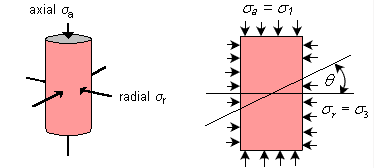To visualise the normal and shear stresses acting on any plane within the soil sample, a graphical representation of stresses called the Mohr circle is obtained by plotting the principal stresses. The sign convention in the construction is to consider compressive stresses as positive and angles measured counter-clockwise also positive.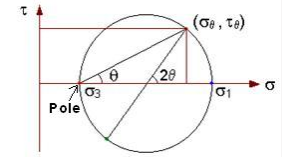Draw a line inclined at angle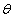with the horizontal through the pole of the Mohr circle so as to intersect the circle. The coordinates of the point of intersection are the normal and shear stresses acting on the plane, which is inclined at anglewithin the soil sample.

Normal stress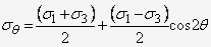Shear stress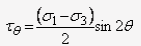The plane inclined at an angle of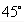to the horizontal has acting on it the maximum shear stress equal to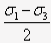, and the normal stress on this plane is equal to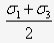.
The plane with the maximum ratio of shear stress to normal stress is inclined at an angle of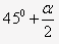to the horizontal, where a is the slope of the line tangent to the Mohr circle and passing through the origin.

Mohr-Coulomb Failure Criterion

When the soil sample has failed, the shear stress on the failure plane defines the shear strength of the soil. Thus, it is necessary to identify the failure plane. Is it the plane on which the maximum shear stress acts, or is it the plane where the ratio of shear stress to normal stress is the maximum?

For the present, it can be assumed that a failure plane exists and it is possible to apply principal stresses and measure them in the laboratory by conducting a triaxial test. Then, the Mohr circle of stress at failure for the sample can be drawn using the known values of the principal stresses.

If data from several tests, carried out on different samples upto failure is available, a series of Mohr circles can be plotted. It is convenient to show only the upper half of the Mohr circle. A line tangential to the Mohr circles can be drawn, and is called the Mohr-Coulomb failure envelope.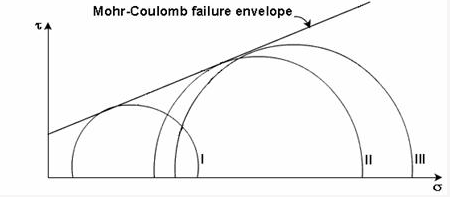If the stress condition for any other soil sample is represented by a Mohr circle that lies below the failure envelope, every plane within the sample experiences a shear stress which is smaller than the shear strength of the sample. Thus, the point of tangency of the envelope to the Mohr circle at failure gives a clue to the determination of the inclination of the failure plane. The orientation of the failure plane can be finally determined by the pole method.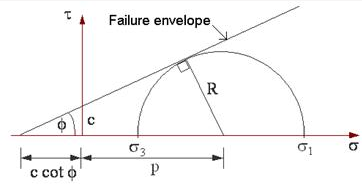The Mohr-Coulomb failure criterion can be written as the equation for the line that represents the failure envelope. The general equation is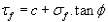Where Tf= shear stress on the failure plane

c = apparent cohesion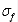= normal stress on the failure plane
f = angle of internal friction

The failure criterion can be expressed in terms of the relationship between the principal stresses. From the geometry of the Mohr circle,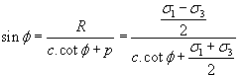Rearranging,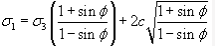where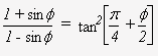Methods of Shear Strength Determination

Direct Shear Test

The test is carried out on a soil sample confined in a metal box of square cross-section which is split horizontally at mid-height. A small clearance is maintained between the two halves of the box.The soil is sheared along a predetermined plane by moving the top half of the box relative to the bottom half. The box is usually square in plan of size
60 mm x 60 mm. A typical shear box is shown.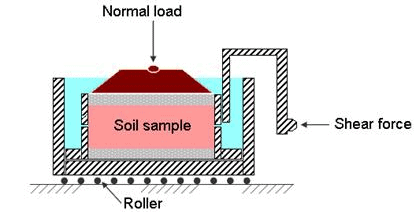If the soil sample is fully or partially saturated, perforated metal plates and porous stones are placed below and above the sample to allow free drainage. If the sample is dry, solid metal plates are used. A load normal to the plane of shearing can be applied to the soil sample through the lid of the box.

Tests on sands and gravels can be performed quickly, and are usually performed dry as it is found that water does not significantly affect the drained strength. For clays, the rate of shearing must be chosen to prevent excess pore pressures building up.

As a vertical normal load is applied to the sample, shear stress is gradually applied horizontally, by causing the two halves of the box to move relative to each other. The shear load is measured together with the corresponding shear displacement. The change of thickness of the sample is also measured.

A number of samples of the soil are tested each under different vertical loads and the value of shear stress at failure is plotted against the normal stress for each test. Provided there is no excess pore water pressure in the soil, the total and effective stresses will be identical. From the stresses at failure, the failure envelope can be obtained.

The test has several advantages:

•  It is easy to test sands and gravels.

•  Large samples can be tested in large shear boxes, as small samples can give misleading results due to imperfections such as fractures and fissures, or may not be truly representative.

•  Samples can be sheared along predetermined planes, when the shear strength along fissures or other selected planes are needed.

The disadvantages of the test include:

•  The failure plane is always horizontal in the test, and this may not be the weakest plane in the sample. Failure of the soil occurs progressively from the edges towards the centre of the sample.

•  There is no provision for measuring pore water pressure in the shear box and so it is not possible to determine effective stresses from undrained tests.

•  The shear box apparatus cannot give reliable undrained strengths because it is impossible to prevent localised drainage away from the shear plane.

Triaxial Test

The triaxial test is carried out in a cell on a cylindrical soil sample having a length to diameter ratio of 2. The usual sizes are 76 mm x 38 mm and 100 mm x 50 mm. Three principal stresses are applied to the soil sample, out of which two are applied water pressure inside the confining cell and are equal. The third principal stress is applied by a loading ram through the top of the cell and is different to the other two principal stresses. A typical triaxial cell is shown.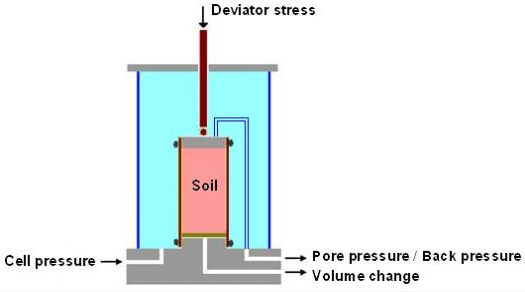The soil sample is placed inside a rubber sheath which is sealed to a top cap and bottom pedestal by rubber O-rings. For tests with pore pressure measurement, porous discs are placed at the bottom, and sometimes at the top of the specimen. Filter paper drains may be provided around the outside of the specimen in order to speed up the consolidation process. Pore pressure generated inside the specimen during testing can be measured by means of pressure transducers.

The triaxial compression test consists of two stages:

First stage: In this, a soil sample is set in the triaxial cell and confining pressure is then applied.

Second stage: In this, additional axial stress (also called deviator stress) is applied which induces shear stresses in the sample. The axial stress is continuously increased until the sample fails.

During both the stages, the applied stresses, axial strain, and pore water pressure or change in sample volume can be measured.

Test Types
There are several test variations, and those used mostly in practice are:

UU (unconsolidated undrained) test: In this, cell pressure is applied without allowing drainage. Then keeping cell pressure constant, deviator stress is increased to failure without drainage.

CU (consolidated undrained) test: In this, drainage is allowed during cell pressure application. Then without allowing further drainage, deviator stress is increased keeping cell pressure constant.

CD (consolidated drained) test: This is similar to CU test except that as deviator stress is increased, drainage is permitted. The rate of loading must be slow enough to ensure no excess pore water pressure develops

In the UU test, if pore water pressure is measured, the test is designated by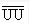.
In the CU test, if pore water pressure is measured in the second stage, the test is symbolized as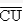Significance of Triaxial Testing
The first stage simulates in the laboratory the in-situ condition that soil at different depths is subjected to different effective stresses. Consolidation will occur if the pore water pressure which develops upon application of confining pressure is allowed to dissipate. Otherwise the effective stress on the soil is the confining pressure (or total stress) minus the pore water pressure which exists in the soil.

During the shearing process, the soil sample experiences axial strain, and either volume change or development of pore water pressure occurs. The magnitude of shear stress acting on different planes in the soil sample is different. When at some strain the sample fails, this limiting shear stress on the failure plane is called the shear strength.

The triaxial test has many advantages over the direct shear test:

•  The soil samples are subjected to uniform stresses and strains.

•  Different combinations of confining and axial stresses can be applied.

•  Drained and undrained tests can be carried out.

•  Pore water pressures can be measured in undrained tests.

•  The complete stress-strain behaviour can be determined.

Offer running on EduRev: Apply code STAYHOME200 to get INR 200 off on our premium plan EduRev Infinity!

## Soil Mechanics

31 videos|48 docs|42 tests

,

,

,

,

,

,

,

,

,

,

,

,

,

,

,

,

,

,

,

,

,

;